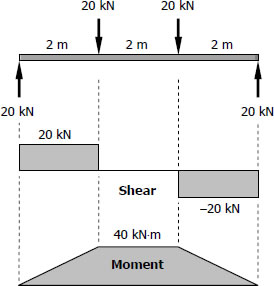# For Girder (G - 1) | Solution to Problem 542

For Girder (G - 1)$S_{live-load} = \dfrac{M}{f_b} = \dfrac{40(1000^2)}{120}$
$S_{live-load} = 333.33 \times 10^3 \, \text{mm}^3$

From Appendix B, Table B-2 Properties of Wide-Flange Sections (W Shapes): SI Units, of text book:

 Designation Section Modulus W200 × 36 342 × 103 mm3 W250 × 33 379 × 103 mm3 W310 × 28 351 × 103 mm3

Consider W310 × 28 with S = 351 × 103 mm3

Checking:$S_{supplied} \ge S_{required} + S_{own-weight}$

$S_{supplied} = 1790 \times 10^3 \, \text{mm}^3$
$S_{required} = 333.33 \times 10^3 \, \text{mm}^3$
$S_{own-weight} = \dfrac{M_{own-weight}}{f_b} = \dfrac{[ \, \frac{1}{8}(274.68)(6^2)](1000)}{120}$
$S_{own-weight} = 10\,300.5 \, \text{mm}^3$

$(1790 \times 10^3) \ge (333.33 \times 10^3) + 10\,300.5$
$(1790 \times 10^3) \ge (343.63 \times 10^3) \,\,$ (ok!)

Use W310 × 28 for G-1. answer

Solution for Beams (B - 1)
Solution for Girder (G - 1)
Solution for Beams (B - 2)
Solution for Beams (B - 3)
Solution for Girders (G - 2)